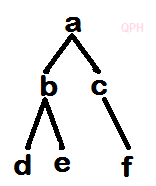# DBATU Database system sem-5 comps

QuestionPapersHub

###### Stay connected :BABASAHEB AMBEDKAR TECHNOLOGICAL UNIVERSITY, LONERE.

Winter Semester Examination – Dec 2019

Branch: B. Tech ( Computer Engineering)-Sem-5

Subject (Subject Code): Database Systems(BTCOC501)

Date: 09/12/2019

Time: 3 Hrs.

Marks: 60

Instructions to the Students :

1. Solve ANY FIVE questions out of the.
2. The level of question/expected answer as per OBE or the Course Outcome on which the question is based is mentioned in front of the.
3. Use of non-programmable scientific calculators is allowed.
4. Assume suitable data wherever necessary & mention it clearly.

Q.1. Attempt any 3(4×3=12).

a) Explain the difference between two-tier & threee-tier architectures. Which is better suited for web applications? Why?

b) Construct an E-R diagram for a car insurance company whose customers own one or more cars each. Each car has associated with it zero to any number of recorded accidents. Each insurance policy coveres one or more cars, and has one or more

c) Suppose the numbers : 50, 15, 62, 5, 20, 58, 91, 3, 8, 37, 60, 24 are inserted in order into an initially empty binary search tree. What is preorder, inorder & postorder transversal sequence of the tree?

d) What is garbage collection? Who will run garbage collection program? When it will be run?

Q.2. Solve all the following  questions(4×3=12).

a) What is sparse matrix? Convert the following sparse matrix.

1 0 0 0

0 -2 11 0

0 0 0 0

0 6 0 5

b) Suppose multidimensional arrays A & B are declared using

A (-1:3) & B (1:5, -3.1).

Find the length of each dimension &  the number of elements in A & B.

p(x)=2x8-5x7+3x2+4 .

d) What is hash data structure?The keys: 32, 18, 23, 2, 3, 44, 5 and 15 are inserted into an initially empty hash table of length 10 with hash function H(Key)= key mod 10 & linear probing is used to resolve collision. What is hash table content after every key insertion?

Q.3. Solve any 3 of the following questions(3×4=12).

a) Give an algorithm to complement binary search with its advantages & disadvantages.

b) Explain the concept of skip list with an example. Give its advantages & disadvantages.

c) Sort the following list using radix sort. Show all the passes neatly:

3 45 7 18 9 4 89 103 11 21

d) Suppose we are sorting an array of eight integers using quick-sort, and we have just finished the first partitioning with the array looking like this:

2, 5, 1, 7, 9, 12, 21, 30

What was the pivot element in the first partition? Also complete the rest of the partitions so that all numbers will be in the ascending order.

Q.4. Solve any 2 of the following (2×6=12).

a) Write an algortihm to insert a new node at the begining of the singly linked list.

b) What is singly circular linked list? Write an algorithm to transverse the list & also enlist different operations performed on it.

c) Write a short note on dynamic storage management. Explain how it is done in C.

Q.5. Solve any 2 (2×6=12).

a) Consider the stack where N=6 memory cells allocated. Suppose initially stack contains A, D, E, F, G (Top of stack). Then the following operatins called in order. Show the stack top & any other situation raised while doing each of the operations.

i) Push (Stack, K)

ii) Pop(Stack, Item)

iii) Push(Stack, L )

iv) Push(Stack, S)

v) Pop(Stack, Item)

vi) Push(Stack, T)

b) What is queue? Write an algorithm to implement insert item into queue using singly linked list.

c) write an algorithm to evaluate postfix expression using stack & execute your algorithm with postfix expression 10, 5, 6, *, +, 8, /.

Show intermediate stack content after each operation.

Q.6. Solve all of the following(4×3=12).

a) Give the characteristics of good algorithm. Also explain how do e analyze the algorithm.

b) Store elements of the given below binary tree using

i) One dimensional array